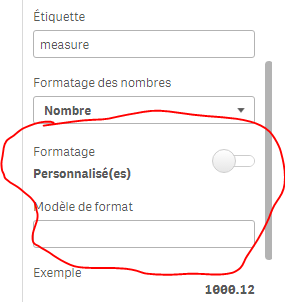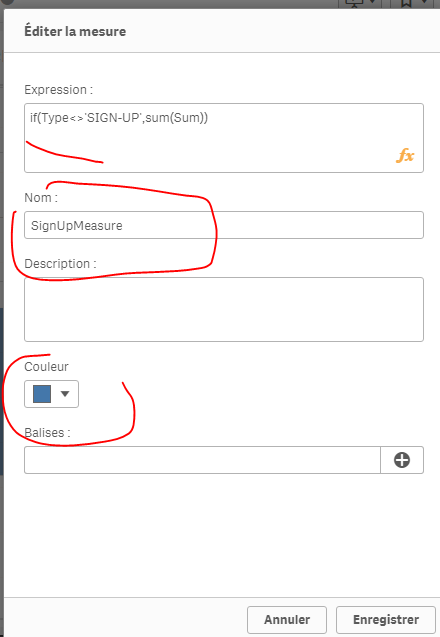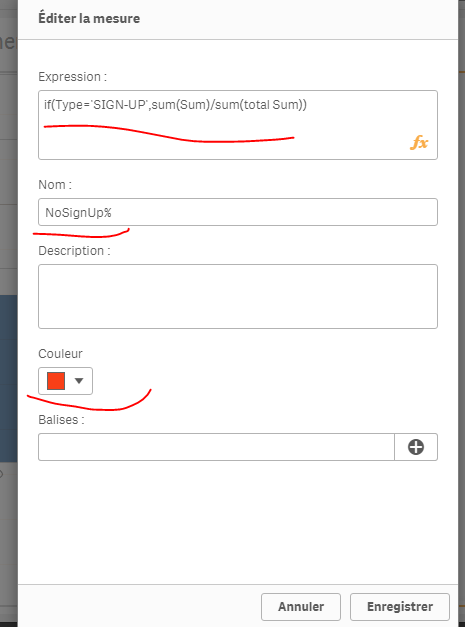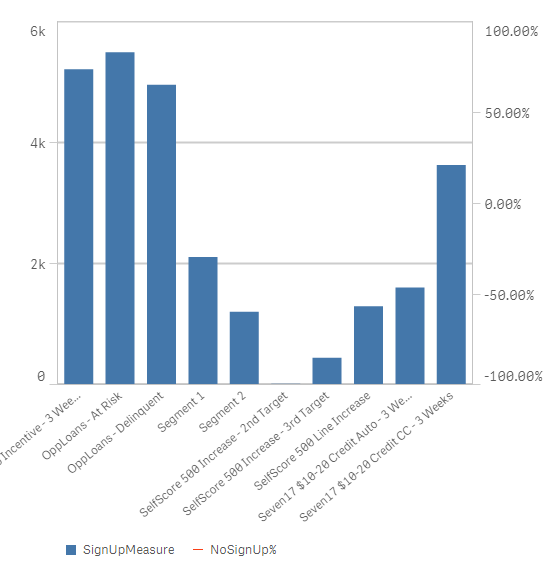# New to Qlik Sense

Discussion board where members can get started with Qlik Sense.Not applicable

## How to change the values in measure as percentage?

Hello All,From the above graph

In delivered emails i have values but in open rate i need those values should be in percentage type as well as in signup rate too.

1 Solution

Accepted Solutions
HighlightedPartner

## Re: How to change the values in measure as percentage?

I found out 2 "solutions"; I don't know if it's appealing enough though..
1st one:

in the same graphic, having some dimensions having sum(Sum) and others as % (but you'll have to know what's the % because I couldn't show the % sign):

Measure :

if(Type='SIGN-UP',num(sum(Sum),'##0,00'), num((sum(Sum)/sum(total Sum))*100,'##0,00%'))result:2) you'll have to use a combined chart and thus, you can't have 2 dimensions in your chart but only ONLY hiearchic dimension :and create 2 master measures:Now, in your chart, use these dimension and measures :

result:I click on open and confirm : I'll have sum(Sum) by segments of type open:see the attached app

You can also have the information of the % for juste the Type OPEN for example:

Type to =Type& ' '&aggr( if(Type='OPEN',num((sum(Sum)/sum(total Sum))*100,'##0,00%')),Type)

Segment_Name to =Segment_Name& ' '&aggr( if(Type='OPEN',num((sum(Sum)/sum(total Sum))*100,'##0,00%')),Segment_Name,Type)

result :13 Replies
Valued Contributor III

## Re: How to change the values in measure as percentage?

I think this is not possible , but if you hover on the bars you will see the Value and SharePartner

## Re: How to change the values in measure as percentage?

Unfortunately, it's not possible to control what's being seen in the pop up in Qlik Sense; the only way to do it is to color by expression, and the expression would be the %; in that case, when you hover on it, you'll see the percentage.

mto‌, in qlikView, we have the possibility to control what's being seen when we hover over a dimension; we could add a measure, make it invisible and it will only appear when we hover over an object.
Is such a possiblity or another direct way (like in tableau) is thought about in the next relase?

Thanks,Not applicable

## Re: How to change the values in measure as percentage?

Hi Goutham

If you looking to convert in % here you are:

1. num((YourExpression), '#,##0%')

2. Number formatting should be Number

3. Formatting should be simple and select 12% from drop downNot applicable

## Re: How to change the values in measure as percentage?

But for Open Rate it should be in percentage, and for delivered emails it must be in valuesPartner

## Re: How to change the values in measure as percentage?

and for the others?
Can you share your app with just this chart on it? I'll try sthingNot applicable

## Re: How to change the values in measure as percentage?

Okay bro,,Not applicable

## Re: How to change the values in measure as percentage?

omarbensalem‌ !

I want Delivered Emails as same(Values) and Open Rate in percentage

HighlightedPartner

## Re: How to change the values in measure as percentage?

I found out 2 "solutions"; I don't know if it's appealing enough though..
1st one:

in the same graphic, having some dimensions having sum(Sum) and others as % (but you'll have to know what's the % because I couldn't show the % sign):

Measure :

if(Type='SIGN-UP',num(sum(Sum),'##0,00'), num((sum(Sum)/sum(total Sum))*100,'##0,00%'))result:2) you'll have to use a combined chart and thus, you can't have 2 dimensions in your chart but only ONLY hiearchic dimension :and create 2 master measures:Now, in your chart, use these dimension and measures :

result:I click on open and confirm : I'll have sum(Sum) by segments of type open:see the attached app

You can also have the information of the % for juste the Type OPEN for example:

Type to =Type& ' '&aggr( if(Type='OPEN',num((sum(Sum)/sum(total Sum))*100,'##0,00%')),Type)

Segment_Name to =Segment_Name& ' '&aggr( if(Type='OPEN',num((sum(Sum)/sum(total Sum))*100,'##0,00%')),Segment_Name,Type)

result :Not applicable

## Re: How to change the values in measure as percentage?

First option i can implement, but i need one more clarification,

I need both OPEN and SIGN-UP should be in percentage

if(Type='OPEN',num((sum(Sum)/sum(total Sum))*100,'##0,00%'), num(sum(Sum),'##0,00'))

Above formula works only for OPEN, but in the same formula can i insert SIGN-Up.

Is it correct=if(Type='OPEN' AND 'SIGN-UP',num((sum(Sum)/sum(total Sum))*100,'##0,00%'), num(sum(Sum),'##0,00'))